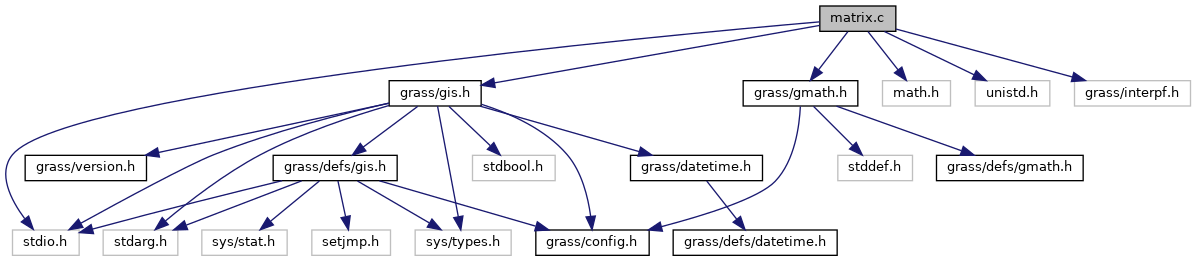GRASS GIS 7 Programmer's Manual  7.9.dev(2021)-e5379bbd7
matrix.c File Reference
`#include <stdio.h>`
`#include <math.h>`
`#include <unistd.h>`
`#include <grass/gis.h>`
`#include <grass/interpf.h>`
`#include <grass/gmath.h>`
Include dependency graph for matrix.c:Go to the source code of this file.

## Functions

int IL_matrix_create (struct interp_params *params, struct triple *points, int n_points, double **matrix, int *indx)

int IL_matrix_create_alloc (struct interp_params *params, struct triple *points, int n_points, double **matrix, int *indx, double *A)
Creates system of linear equations from interpolated points. More...

## ◆ IL_matrix_create()

 int IL_matrix_create ( struct interp_params * params, struct triple * points, int n_points, double ** matrix, int * indx )

Definition at line 39 of file matrix.c.

## ◆ IL_matrix_create_alloc()

 int IL_matrix_create_alloc ( struct interp_params * params, struct triple * points, int n_points, double ** matrix, int * indx, double * A )

Creates system of linear equations from interpolated points.

Creates system of linear equations represented by matrix using given points and interpolating function interp()

Parameters
 params struct interp_params * points points for interpolation as struct triple n_points number of points [out] matrix the matrix indx
Returns
-1 on failure, 1 on success

Definition at line 72 of file matrix.c.